flat assembler
Message board for the users of flat assembler.Index > Main > Compute 10^n/10 using only 1 register with 2 instructions Goto page 1, 2, 3  Next
Author
 Threadrevolution When all else fails, read the source Joined: 24 Aug 2004 Posts: 17668 Location: In your JS exploiting you and your system revolution When computing decreasing powers of 10 there is a trick we can use to get the result without using division and without using any other registers. Code: imul ebx,0xcccccccd shr ebx,1 Here we use the familiar 8/10 * 2^32 constant for dividing by 10 with a multiply. But we throw away the result and only keep the "useless" low order bits. Well in this case the low order bits has our desired result once we halve it. This also works in 64 bit ... Code: imul rbx,0xcccccccccccccccd ;??? shr rbx,1 ... BUT we can't encode that value directly as an immediate in x86-64. We either have to have a register with the value, or use a memory location. Note: That this is only valid for powers of 10. Other numbers probably don't work (I haven't investigated all the other number patterns). And especially the input value of 1 gives the wrong result. Anyhow, I found this useful in a number conversion function when I was short of registers.27 Jan 2019, 12:11
 Tomasz Grysztar Joined: 16 Jun 2003 Posts: 7797 Location: Kraków, Poland Tomasz Grysztar If you look at my post about division by multiplication you may find a link to a very old discussion on the other message board where I wrote about such trick: Tomasz Grysztar wrote:If you want to divide by the odd number, and you know the number you want to divide is divisible without remainder, you can do this by multiplying by "magic" number and take the LOW half of the result, without need to do any shifts etc. This approach is based on the theory of 2-adic numbers. (...) Let me explain how it applies to your case. In the field of 2-adic numbers the representation of number 1/5 is an infinite sequence of digits: ...00110011001100110011001101. if we group them in fours and convert to hexadecimal, this is: ...CCCCCCCCCD. Now, in general when you multiply any p-adic numbers, the low n digits of product only depend on the low n digits of factors. So if you only take low n digits of a rational number like 1/5 and multiply another number by it, the low n digits of the result are going to be exactly the same as in actual product of 1/5 and the other number. Therefore this method should always give a perfect result when the other number is divisible by 5, because then the result is an integer and everything above the low n digits is (in 2-adic case) an infinite sequence of 0s or 1s (depending whether the integer was positive or negative). However, when the number is not divisible by 5, the result is a rational number with a more complex infinite expansion, for example: 9*...CCCCCCCD. = ...33333335. The low digits of 2-adic representation of 9/5 do not resemble a small integer at all.27 Jan 2019, 14:18
 revolution When all else fails, read the source Joined: 24 Aug 2004 Posts: 17668 Location: In your JS exploiting you and your system revolution I'm still trying to understand the whole 2-adic thing. The high-order bits I can understand how that works, but the low order bits ... they are still a mystery to me about what happens there.27 Jan 2019, 15:15
 Tomasz Grysztar Joined: 16 Jun 2003 Posts: 7797 Location: Kraków, Poland Tomasz Grysztar Let me try to explain it as simply as I can. For certain you are already familiar with positional numeral systems like decimal, binary, hexadecimal, etc. They can represent any positive number in form:whereWe usually write down such representation as a sequence with the highest power to the left: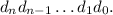Now, p-adic numbers have analogous representations, but they are potentially infinite:Normally this kind of infinite sum would be divergent. But when the base is a prime number, it is possible to define such function of distance between any two numbers (based on p-adic norm) that these sums become convergent (with p-adic norm the powers of p are closer and closer to zero the higher the exponent is). Since this only works for prime numbers, we use "p" to denote such base. 2 is prime, so we can have 2-adic numbers. They are like binary numbers, but including infinite ones. As we usually write higher powers on the left, we have to append an infinite number of digits on the left side. Any regular positive integer has a representation that just has infinitely many zero digits appended on the left: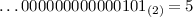What about the other sequences? Let's consider a number that has all binary digits set to 1: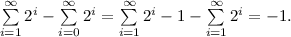So this is a representation of a negative number. In fact, all negative numbers are going to have representations that have all digits set to 1 starting at some point. (Note that the calculation we did would normally be invalid, but it is correct under a 2-adic metric, all these sums are convergent then). It is possible to add and subtract these numbers just like you would do it with finite ones (with carry), except that here you have to repeat carrying till infinity. If you add one to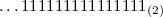, the carry is going to repeat endlessly, leaving just zero digits behind. It confirms that this number is the same as minus one. This nicely relates to the two's complement interpretation of binary numbers in computer architectures. Any such number can be seen as a lowest n digits of a 2-adic number with assumption that all higher digits are the same as the highest digit (called "sign bit") of these n. For example, with 8-bit representations: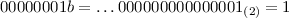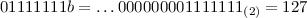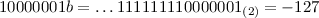The two's complement arithmetic is a kind of projection of a more general 2-adic arithmetic, and this is what makes it work so well. There are still many other infinite sequences possible. Let's take a look at one that has 0s and 1s interleaved: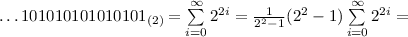This time we got a fractional number. We can verify this through multiplication. Just like addition and subtraction, multiplication of p-adic numbers (which under the hood is a multiplication of infinite series) can be done in the same manner as classic long multiplication, except that it needs to be prolonged infinitely to the left: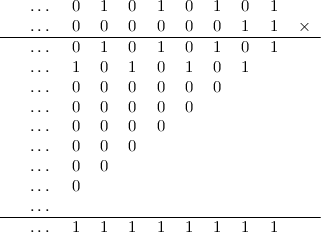This one turned out quite simple, because number 3 has just two bits set, so multiplication by 3 ends up being just a sum of a number and its copy multiplied by 2 (that is: shifted one digit to the left). And thus we have verified that the representation of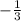multiplied by 3 indeed gives -1 as a result. I encourage to play with this a little, for example subtractfrom 0 to see whatlooks like and then multiply that by 3, which should result in plain 1. Looking at this long multiplication algorithm, we see that low n digits of result only depend on the low n bits of the factors. So, for example, when we know that the result of multiplication is going to be small positive integer, we only need to compute the rightmost digits, as we know that the remaining ones to the left are going to be an infinite chain of zeros. Every rational number with an odd denominator has a periodic 2-adic representation. What about even denominators? Dividing a number by 2 is equivalent to shifting its representation right by on digit and we may end up shifting some digits to the negative powers. This is analogous to formal Laurent series. For example: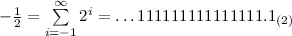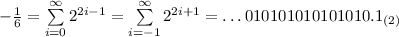________________________________________________________________________ Now, how can we find 2-adic representation of any fraction? Or p-adic in general? For a number in form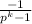we already know the drill: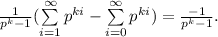This gives us, for example: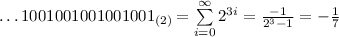The value of k gives us the length of a period, and the numbers of this form have a representation of mostly zeros, with 1 every k digits. Multiplying by p is the same as shifting left, so numbers like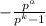also have a very similar simple form: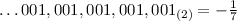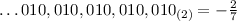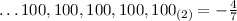This kind of number has a period k, with just a single 1 digit repeated over this period. The ones that have numerator smaller than denominator do not overlap with each other. We can therefore split a numerator into parts according to its positional representation, and the terms we get can be easily merged with each other. For example, let's take 5 = 101b = 4 + 1: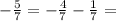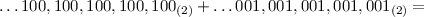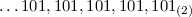This demonstrates that negative fractions of such form (with denominator equal to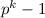for some k) have a periodic structure and the repeated pattern has the same digits as a representation of numerator (as long as it fits within the number of digits provided by the length of the period, which is true when numerator is smaller than denominator). To make positive fractions out of these negative ones, it is enough to add 1: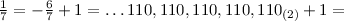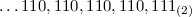If we have a denominator n that is not in such nice form, but at least is not divisible by p, we just need to find k such thatis a multiple of n. As p and n are relatively prime, such k must exist (it follows from Chinese remainder theorem). In particular, when n is also a prime, such exponent may be quickly obtained with aid of Fermat's little theorem, which states that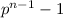is then a multiple of n. For example, with n=5 (and p=2) we get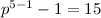, which indeed is a multiple of 5. Then to convert a fraction we just need to multiply numerator and denominator with appropriate value (in this case 3) to obtain denominator of form: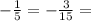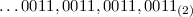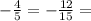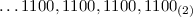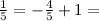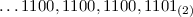Finally, if a denominator is divisible by p, we should factorize it into a power of p and a number not divisible by p: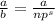. Then, after finding a representation of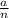, we shift it right by s digits: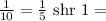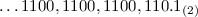This is a Laurent-series-like representation, it has a digit corresponding to a negative power of 2. Last edited by Tomasz Grysztar on 14 Feb 2019, 09:21; edited 7 times in total27 Jan 2019, 17:15
 revolution When all else fails, read the source Joined: 24 Aug 2004 Posts: 17668 Location: In your JS exploiting you and your system revolution So the 2-adic representation of 1/5 (hex ...cccccccc) can be multiplied by any other number with a factor of 5 and we always get a whole number result. Let's try it out 555555555₁₀ × cccccccd₁₆ = {......}069F6BC7₁₆ which truncates to 111111111₁₀ It works. But I have to make the value cccccccd₁₆27 Jan 2019, 17:39
 revolution When all else fails, read the source Joined: 24 Aug 2004 Posts: 17668 Location: In your JS exploiting you and your system revolution Can we also use that to check for divisibility by 5 by multiplying the result by 5 and see if it equals the original number? Edit: Of course not, we will get back the same result since 5 * 1/5. Doh. Edit2: Brute force to the rescue Code:format ELF executable 0 entry main SYS_EXIT = 1 SYS_WRITE = 4 STD_OUTPUT = 1 segment executable main: xor eax,eax .loop: mov ecx,eax imul ecx,0xcccccccd imul ecx,5 cmp eax,ecx jne .failed inc eax jnz .loop mov ecx,success mov edx,success.len jmp .close .failed: mov edx,eax mov ecx,fail_at call write_hex mov ecx,failure mov edx,failure.len .close: mov eax,SYS_WRITE mov ebx,STD_OUTPUT int 0x80 mov eax,SYS_EXIT xor ebx,ebx int 0x80 write_hex: ;ecx = address ;edx = value .next_nibble: mov eax,edx shr eax,28 cmp al,10 sbb al,0x69 das mov [ecx],al inc ecx shl edx,4 jnz .next_nibble retn segment readable writeable failure: db 'Failed at 0x' fail_at db '00000000',10 failure.len = $- failure success: db 'All values passed okay',10 success.len =$ - success  Quote:All values passed okay I'm not going to try this with the full 64-bits!27 Jan 2019, 17:42
 Tomasz Grysztar Joined: 16 Jun 2003 Posts: 7797 Location: Kraków, Poland Tomasz Grysztar revolution wrote:It works. But I have to make the value cccccccd₁₆ Yes, that's what I wrote: Tomasz Grysztar wrote:1/5 is an infinite sequence of digits: ...00110011001100110011001101. if we group them in fours and convert to hexadecimal, this is: ...CCCCCCCCCD. As for 2-adic ...CCCCCCCC, it is a representation of -4/5. Please wait while I update my above post with additional information how to convert various rational numbers to 2-adic forms.27 Jan 2019, 18:18
 Tomasz Grysztar Joined: 16 Jun 2003 Posts: 7797 Location: Kraków, Poland Tomasz Grysztar I have edited my long post above and added a section explaining how to obtain a 2-adic representation of any rational number. Because numbers that have denominator divisible by 2 have representations that include negative powers of 2, only fractions with an odd denominator can be used in the form of their low 32/64 digits for computations like yours. This is why to divide by 10 you have to use 1/5 and shift. As long as you know that your number is exactly divisible by an odd denominator, you can use the 2-adic reciprocal safely. It is not hard to prove that the low 32/64 bits of the result are then going to be correct (even for negative numbers!). I believe that even a simplified explanation that I provided here should be enough of a foundation for a proof.27 Jan 2019, 19:45
 Tomasz Grysztar Joined: 16 Jun 2003 Posts: 7797 Location: Kraków, Poland Tomasz Grysztar A bonus question: do you see a relation between this topic and the fact that x86 has "IMUL dest,src" but no "MUL dest,src"?27 Jan 2019, 20:20
 revolution When all else fails, read the source Joined: 24 Aug 2004 Posts: 17668 Location: In your JS exploiting you and your system revolution So the following bit patterns for divisors should always give correct results. Code:0x00000001 0x00000003 0x00000005 <--- the one I used above 0x0000000f 0x00000011 0x00000033 0x00000055 0x000000ff 0x00000101 0x00000303 0x00000505 0x00000f0f 0x00001111 0x00003333 0x00005555 0x0000ffff 0x00010001 0x00030003 0x00050005 0x000f000f 0x00110011 0x00330033 0x00550055 0x00ff00ff 0x01010101 0x03030303 0x05050505 0x0f0f0f0f 0x11111111 0x33333333 0x55555555 0xffffffff27 Jan 2019, 20:27
 revolution When all else fails, read the source Joined: 24 Aug 2004 Posts: 17668 Location: In your JS exploiting you and your system revolution Tomasz Grysztar wrote:A bonus question: do you see a relation between this topic and the fact that x86 has "IMUL dest,src" but no "MUL dest,src"? Yes, I do now.27 Jan 2019, 20:28
 Tomasz Grysztar Joined: 16 Jun 2003 Posts: 7797 Location: Kraków, Poland Tomasz Grysztar revolution wrote:So the following bit patterns for divisors should always give correct results (...) Not just these ones, it should work for any odd number. A 2-adic reciprocal of an odd integer is always a 2-adic integer (that is: a 2-adic number with no negative powers of 2). This is something that is normally proven with help of p-adic valuation, but since I already gave an exact algorithm for obtaining a 2-adic reciprocal of an odd number, analyzing this algorithm is enough to prove it.27 Jan 2019, 20:31
 revolution When all else fails, read the source Joined: 24 Aug 2004 Posts: 17668 Location: In your JS exploiting you and your system revolution Tomasz Grysztar wrote: revolution wrote:So the following bit patterns for divisors should always give correct results (...) Not just these ones, it should work for any odd number. A 2-adic reciprocal of an odd integer is always a 2-adic integer (that is: a 2-adic number with no negative powers of 2). I meant for 32-bit integers. The period of the divisor needs to be a factor of 32 or we can't use it to find divisors unless we have 36-bit (or some other length) CPUs.27 Jan 2019, 20:36
 Tomasz Grysztar Joined: 16 Jun 2003 Posts: 7797 Location: Kraków, Poland Tomasz Grysztar revolution wrote:I meant for 32-bit integers. The period of the divisor needs to be a factor of 32 or we can't use it to find divisors unless we have 36-bit (or some other length) CPUs. Oh, that is you want to find divisor at run-time. But you are more likely to have the reciprocal value pre-computed and then this is should not be a concern. Also, I think there are other possible algorithms to obtain a 2-adic reciprocal. I have chosen this one because I believe it shows nicely various interactions and allows to quickly get a better grasp of what is going on with these patterns.27 Jan 2019, 20:41
 revolution When all else fails, read the source Joined: 24 Aug 2004 Posts: 17668 Location: In your JS exploiting you and your system revolution Tomasz Grysztar wrote:Oh, that is you want to find divisor at run-time Yes. I look for a programming usage from the discussion. So we know from above that 7 can't be used in this way on 32-bit CPUs. Which means that if I have a problem where I need to divide by 7 I already know I have to find some other method.27 Jan 2019, 20:53
 Tomasz Grysztar Joined: 16 Jun 2003 Posts: 7797 Location: Kraków, Poland Tomasz Grysztar revolution wrote: Tomasz Grysztar wrote:Oh, that is you want to find divisor at run-time Yes. I look for a programming usage from the discussion. So we know from above that 7 can't be used in this way on 32-bit CPUs. Which means that if I have a problem where I need to divide by 7 I already know I have to find some other method. Wait, why do you think you would not be able to divide by 7?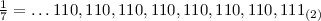This in hexadecimal form becomes: ...DB6DB6DB6DB7h If you cut it down to the lowest 32 bits, you get 0B6DB6DB7h. Please run your tests and see that as long as dividend is a multiple of 7, multiplication by this reciprocal gives the right result. A 2-adic reciprocal of any odd number, cut down to its lowest 32 digits, is going to work correctly for all 32-bit inputs divisible by that odd number.27 Jan 2019, 21:01
 revolution When all else fails, read the source Joined: 24 Aug 2004 Posts: 17668 Location: In your JS exploiting you and your system revolution Tomasz Grysztar wrote: revolution wrote: Tomasz Grysztar wrote:Oh, that is you want to find divisor at run-time Yes. I look for a programming usage from the discussion. So we know from above that 7 can't be used in this way on 32-bit CPUs. Which means that if I have a problem where I need to divide by 7 I already know I have to find some other method. Wait, why do you think you would not be able to divide by 7?This in hexadecimal form becomes: ...DB6DB6DB6DB7h If you cut it down to the lowest 32 bits, you get 0B6DB6DB7h. Please run your tests and see that as long as dividend is a multiple of 7, multiplication by this reciprocal gives the right result. A 2-adic reciprocal of any odd number, cut down to its lowest 32 digits, is going to work correctly for all 32-bit inputs divisible by that odd number. Oh, so the computation of the constant is different. I was thinking that it would be 2^32 * 6 / 7 = 0xDB6DB6DB (or 0xDB6DB6DC).28 Jan 2019, 04:10
 revolution When all else fails, read the source Joined: 24 Aug 2004 Posts: 17668 Location: In your JS exploiting you and your system revolution So if -1/3 = ...55555555₁₆ We can square that ...55555555₁₆^2 = ...e38e38e38e39₁₆ = +1/9 Therefore to divide by 9 we can use this: Code: imul ebx,0x38E38E39 ;divide multiples of 9 by 9 So how to compute the value 0x38E38E39? I'm still foggy on that procedure. Do I need to know the factors of 9 ahead of time? If just take 1/9 = 0x0.1C71C71C71C71C7...) I need to double it to get 0x0.38E38E38E38E... In the case of 1/7 = 0x0.249249249249... I need to multiply by 5 to get 0x0.B6DB6DB6D...28 Jan 2019, 07:01
 Tomasz Grysztar Joined: 16 Jun 2003 Posts: 7797 Location: Kraków, Poland Tomasz Grysztar I have shown the entire procedure in the second half of my edited post (I added that part later).was among the examples there. For denominator 9, you first need to find its multiple that has form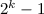. One that works is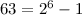. 2-adic representation of a number with such denominator is going to have a period of 6: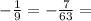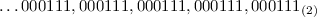(the digits within a single block are the same as binary representation of 7)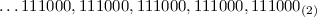(because 56 = 111000b, but it is also just a simple shift left by 3 digits)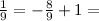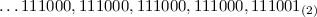(with hex digits it is ...E38,E38,E38,E39)28 Jan 2019, 07:57
 revolution When all else fails, read the source Joined: 24 Aug 2004 Posts: 17668 Location: In your JS exploiting you and your system revolution Tomasz Grysztar wrote:For denominator 9, you first need to find its multiple that has form. One that works is. 2-adic representation of a number with such denominator is going to have a period of 6: This is the missing piece. We have to find the period first and then we can shift the result some number of bits to align the period with the bit 0. Period of 7 = 3 Period of 9 = 6 Period of 11 = 10 For 7: divisor = 1 + 2^(32 - 32 mod period) * 6 / 7 = 0x36DB6DB7 Oops, fails For 9: divisor = 1 + 2^(32 - 32 mod period) * 8 / 9 = 0x38E38E39 For 11: divisor = 1 + 2^(32 - 32 mod period) * 10 / 11 = 0x3A2E8BA3 Oops, fails. We lost too many bits a the top. Try again For 11: divisor = 1 + 2^(32 + period - 32 mod period) * 10 / 11 = 0x(E8)BA2E8BA3 Works. But it needs more than 32 bits to compute the value.However looking at the result we can just set the top bit manually and we get a working value.28 Jan 2019, 09:34
 Display posts from previous: All Posts1 Day7 Days2 Weeks1 Month3 Months6 Months1 Year Oldest FirstNewest FirstJump to: Select a forum Official----------------AssemblyPeripheria General----------------MainTutorials and ExamplesDOSWindowsLinuxUnixMenuetOS Specific----------------MacroinstructionsOS ConstructionIDE DevelopmentProjects and IdeasNon-x86 architecturesHigh Level LanguagesProgramming Language DesignCompiler Internals Other----------------FeedbackHeapTest Area
Goto page 1, 2, 3  Next

Forum Rules:
 You cannot post new topics in this forumYou cannot reply to topics in this forumYou cannot edit your posts in this forumYou cannot delete your posts in this forumYou cannot vote in polls in this forumYou cannot attach files in this forumYou can download files in this forum